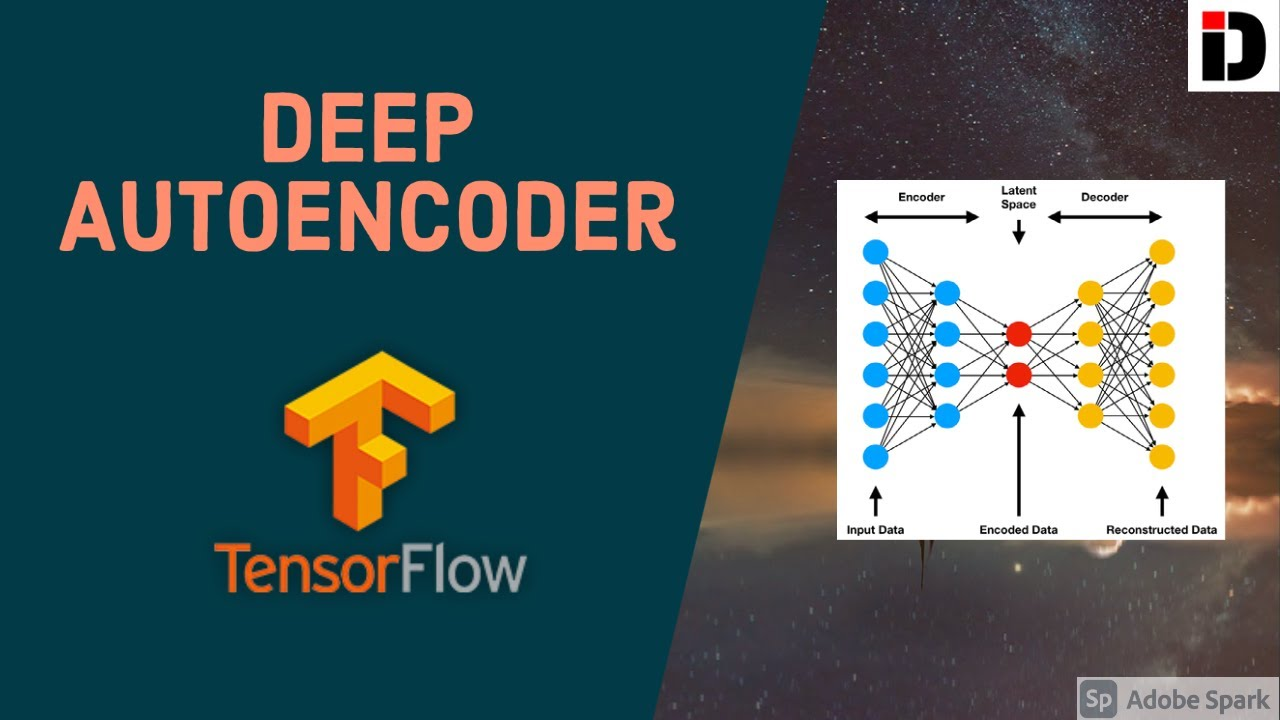1610994900

# Deep Autoencoder in TensorFlow 2.0 (Keras) | Autoencoders Explained

In this video, we are going to dive into the world of Autoencoders and build a Deep Autoencoders in TensorFlow using Keras API. An Autoencoders is a class of neural network for which the input and the output are same. This works by compressing the input into a latent space representation and then reconstructing the output from this representation.

#tensorflow #keras

## Buddha Community1610994900

## Deep Autoencoder in TensorFlow 2.0 (Keras) | Autoencoders Explained

In this video, we are going to dive into the world of Autoencoders and build a Deep Autoencoders in TensorFlow using Keras API. An Autoencoders is a class of neural network for which the input and the output are same. This works by compressing the input into a latent space representation and then reconstructing the output from this representation.

#tensorflow #keras1594525380

## Keras vs. Tensorflow - Difference Between Tensorflow and Keras

Keras and Tensorflow are two very popular deep learning frameworks. Deep Learning practitioners most widely use Keras and Tensorflow. Both of these frameworks have large community support. Both of these frameworks capture a major fraction of deep learning production.

Which framework is better for us then?

This blog will be focusing on Keras Vs Tensorflow. There are some differences between Keras and Tensorflow, which will help you choose between the two. We will provide you better insights on both these frameworks.

## What is Keras?

Keras is a high-level API built on the top of a backend engine. The backend engine may be either TensorFlow, theano, or CNTK. It provides the ease to build neural networks without worrying about the backend implementation of tensors and optimization methods.

Fast prototyping allows for more experiments. Using Keras developers can convert their algorithms into results in less time. It provides an abstraction overs lower level computations.

### Major Applications of Keras

• The performance of Keras is smooth on both CPU and GPU.
• Keras provides modularity, flexibility to code, extensibility, and has an adaptation for innovation and research.
• The pythonic nature of Keras makes it easy to explore and debug the code.

## What is Tensorflow?

Tensorflow is a tool designed by Google for the deep learning developer community. The aim of TensorFlow was to make deep learning applications accessible to the people. It is an open-source library available on Github. It is one of the most famous libraries to experiment with deep learning. The popularity of TensorFlow is because of the ease of building and deployment of neural net models.

Major area of focus here is numerical computation. It was built keeping the processing computation power in mind. Therefore we can run TensorFlow applications on almost kind of computer.

### Major applications of Tensorflow

• From mobiles to embedded devices and distributed servers Tensorflow runs on all the platforms.
• Tensorflow is the enterprise of solving real-world and real-time problems like image analysis, robotics, generating data, and NLP.
• Developers are implementing tools for translation languages and the detection of skin cancers using Tensorflow.
• Major projects using TensorFlow are Google translate, video detection, image recognition.

#keras tutorials #keras vs tensorflow #keras #tensorflow1619701680

## DEEP LEARNING WITH TENSORFLOW 2.0 Machine Learning framework

TensorFlow is the Machine Learning framework by Google. It is primarily used for Deep Learning related tasks and seamlessly integrates with other Google APIs as well. TensorFlow is clearly one of the most used libraries for Deep Learning in the industry right now and totally worth learning!

By the end of this tutorial, you will have knowledge of the following.

• What is TensorFlow?
• What is new in TF 2.0?
• TensorFlow vs Keras
• Installing TensorFlow
• Image Classifier in TensorFlow

#artificial intelligence #deep learning #deep learning with tensorflow 2.0 #tensorflow2.01620256440

## DEEP LEARNING WITH TENSORFLOW 2.0 Machine Learning framework

TensorFlow is the Machine Learning framework by Google. It is primarily used for Deep Learning related tasks and seamlessly integrates with other Google APIs as well. TensorFlow is clearly one of the most used libraries for Deep Learning in the industry right now and totally worth learning!

By the end of this tutorial, you will have knowledge of the following.

• What is TensorFlow?
• What is new in TF 2.0?
• TensorFlow vs Keras
• Installing TensorFlow
• Image Classifier in TensorFlow

### What Is TensorFlow?

TensorFlow started as an Open-Source Deep Learning library by Google and now is a complete framework for end to end Machine Learning processes. You might be wondering why Google chose this name and what does “Tensor” mean.

#artificial intelligence #deep learning #deep learning with tensorflow 2.0 #tensorflow2.01598682060

## Why Deep Learning is Becoming so Popular? | Deep Learning Tutorial (TensorFlow 2.0, Keras & Python)

This video explains four reasons why deep learning has become so popular in past few years.
In this deep learning tutorial python, I will cover following things in this video,

• 00:00 Introduction
• 00:24 Data growth